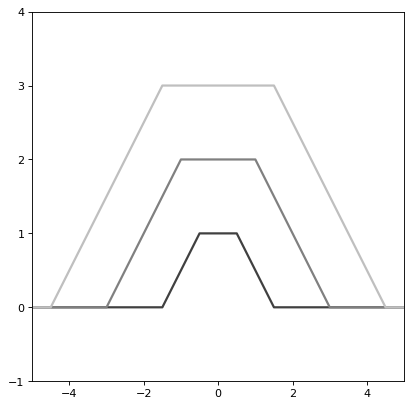Trapezoid1D¶

class astropy.modeling.functional_models.Trapezoid1D(amplitude=1, x_0=0, width=1, slope=1, **kwargs)[source]

One dimensional Trapezoid model.

Parameters
amplitudefloat

Amplitude of the trapezoid

x_0float

Center position of the trapezoid

widthfloat

Width of the constant part of the trapezoid.

slopefloat

Slope of the tails of the trapezoid

Other Parameters
fixeda dict, optional

A dictionary {parameter_name: boolean} of parameters to not be varied during fitting. True means the parameter is held fixed. Alternatively the fixed property of a parameter may be used.

tieddict, optional

A dictionary {parameter_name: callable} of parameters which are linked to some other parameter. The dictionary values are callables providing the linking relationship. Alternatively the tied property of a parameter may be used.

boundsdict, optional

A dictionary {parameter_name: value} of lower and upper bounds of parameters. Keys are parameter names. Values are a list or a tuple of length 2 giving the desired range for the parameter. Alternatively, the min and max properties of a parameter may be used.

eqconslist, optional

A list of functions of length n such that eqcons[j](x0,*args) == 0.0 in a successfully optimized problem.

ineqconslist, optional

A list of functions of length n such that ieqcons[j](x0,*args) >= 0.0 is a successfully optimized problem.

Examples

import numpy as np
import matplotlib.pyplot as plt

from astropy.modeling.models import Trapezoid1D

plt.figure()
s1 = Trapezoid1D()
r = np.arange(-5, 5, .01)

for factor in range(1, 4):
s1.amplitude = factor
s1.width = factor
plt.plot(r, s1(r), color=str(0.25 * factor), lw=2)

plt.axis([-5, 5, -1, 4])
plt.show()

(png, svg, pdf)Attributes Summary

 amplitude input_units This property is used to indicate what units or sets of units the evaluate method expects, and returns a dictionary mapping inputs to units (or None if any units are accepted). param_names Names of the parameters that describe models of this type. slope width x_0

Methods Summary

 evaluate(x, amplitude, x_0, width, slope) One dimensional Trapezoid model function

Attributes Documentation

amplitude = Parameter('amplitude', value=1.0)
input_units
param_names = ('amplitude', 'x_0', 'width', 'slope')

Names of the parameters that describe models of this type.

The parameters in this tuple are in the same order they should be passed in when initializing a model of a specific type. Some types of models, such as polynomial models, have a different number of parameters depending on some other property of the model, such as the degree.

When defining a custom model class the value of this attribute is automatically set by the Parameter attributes defined in the class body.

slope = Parameter('slope', value=1.0)
width = Parameter('width', value=1.0)
x_0 = Parameter('x_0', value=0.0)

Methods Documentation

static evaluate(x, amplitude, x_0, width, slope)[source]

One dimensional Trapezoid model function